Note: The other languages of the website are Google-translated. Back to English

## Excel Formula: Extract first and last name from e-mail address

This tutorial introduces the formulas to extract first name and last name from given email addresses into separate cells as below screenshot shown, also, it provides the example to explain the arguments and parts of the formulas.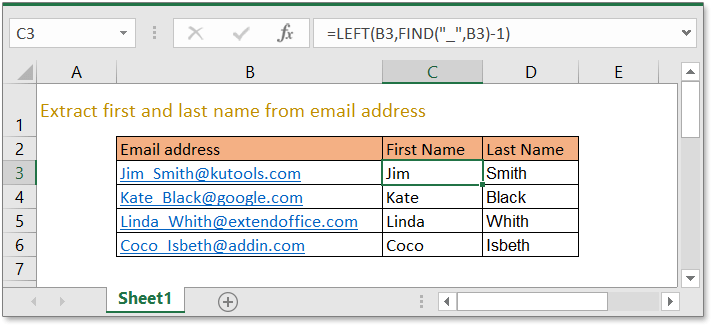### Extract first name

Generic formula:

Arguments

 E_address: the email address you want to extract the first name. Separator: the delimiter between first name and last name.

How this formula work

To extract first name from the email address in cell B3, please use below formula:

 =LEFT(B3,FIND("_",B3)-1)

Press Enter key.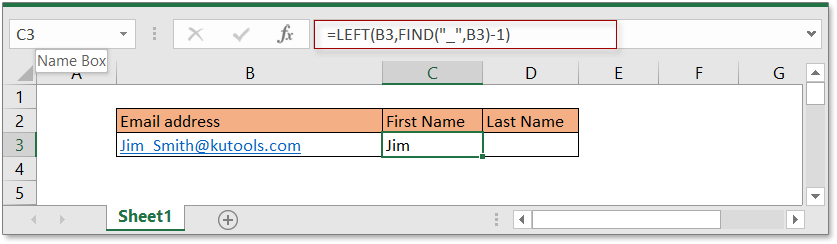Explanation

FIND function: to find the start position of a character or text in a given text string. The formula FIND("_",B3) finds the position of character “_” in the cell B3, returns 4.

LEFT function: extract text from left side of the given text string based on the given text length. Formula LEFT(B3,FIND("_",B3)-1) can be seen as LEFT(B3,4-1), extract first 3 characters from the text in cell B3.

### Extract last name

Generic formula

Argument

 E_address: the email address you want to extract the first name. Separator: the delimiter between first name and last name.

How this formula work

To extract last name from the email address in cell B3, please use below formula:

 =LEFT(RIGHT(B3,LEN(B3)-FIND("_",B3)),FIND("@",RIGHT(B3,LEN(B3)-FIND("_",B3)))-1)

Press Enter key.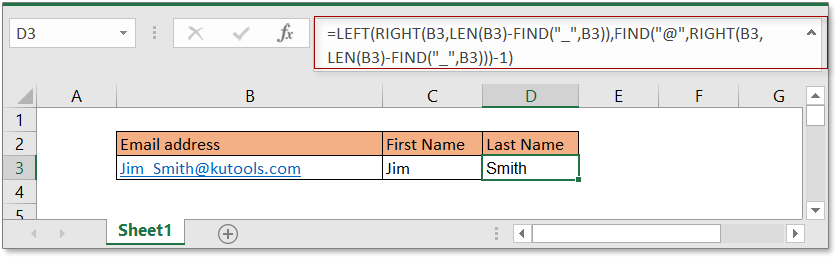Explanation

FIND function: to find the start position of a character or text in a given text string.

LEN function: count the number of a text string.

RIGHT function: to extract text from right side.

The formula RIGHT(B3,LEN(B3)-FIND("_",B3)) can be seen as:
=RIGHT(B3,LEN(B3)-4)
=RIGHT(B3,17)
="Smith@kutools.com"

Then FIND("@",RIGHT(B3,LEN(B3)-FIND("_",B3))) can be seen as
=FIND("@","Smith@kutools.com")
="6"

LEFT function: extract text from left side of the given text string based on the given text length. The formula LEFT(RIGHT(B3,LEN(B3)-FIND("_",B3)),FIND("@",RIGHT(B3,LEN(B3)-FIND("_",B3)))-1) can be seen as these:
=LEFT(RIGHT(B3,LEN(B3)-FIND("_",B3)),6-1)
=LEFT("Smith@kutools.com",5)
="Smith"

Remark

If you want to extract first and last name with first letter uppercase, you can add the PROPER function in the front of above formulas, such as:

=PROPER(LEFT(B3,FIND("_",B3)-1))
=PROPER(LEFT(RIGHT(B3,LEN(B3)-FIND("_",B3)),FIND("@",RIGHT(B3,LEN(B3)-FIND("_",B3)))-1))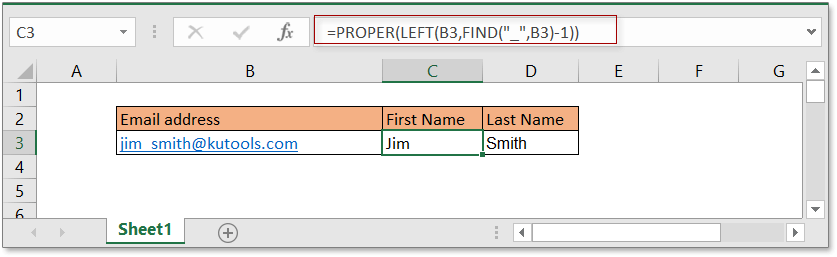### Sample File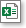Click to download sample file

### The Best Office Productivity Tools

#### Kutools for Excel - Helps You To Stand Out From Crowd

Would you like to complete your daily work quickly and perfectly? Kutools for Excel brings 300 powerful advanced features (Combine workbooks, sum by color, split cell contents, convert date, and so on...) and save 80% time for you.

• Designed for 1500 work scenarios, helps you solve 80% Excel problems.
• Reduce thousands of keyboard and mouse clicks every day, relieve your tired eyes and hands.
• Become an Excel expert in 3 minutes. No longer need to remember any painful formulas and VBA codes.
• 30-day unlimited free trial. 60-day money back guarantee. Free upgrade and support for 2 years.#### Office Tab - Enable Tabbed Reading and Editing in Microsoft Office (include Excel)

• One second to switch between dozens of open documents!
• Reduce hundreds of mouse clicks for you every day, say goodbye to mouse hand.
• Increases your productivity by 50% when viewing and editing multiple documents.
• Brings Efficient Tabs to Office (include Excel), Just Like Chrome, Firefox, And New Internet Explorer.Rated 5 out of 5 · 1 ratings
This comment was minimized by the moderator on the site
Thank you very much for this article. I have another question regarding these formula.
- Say I have these scenarios - I want to separate FNAME & LNAME from this email ann.bee@yahoo.com
With this command =PROPER(LEFT(A4,FIND("_",A4)-1)); I will be able to produce Ann Bee, but this sign "__ must be changed to "." ,

How do I fix this when this happens say I have abee@yahoo.com - this previous command will produce abee@yahoo as the first name when I am looking to achieve Abee....please can you help.
This comment was minimized by the moderator on the site
I realized using the @ within the command also helped but did not isolate the first and last name where applicable.
=PROPER(LEFT(A4,FIND("@",A4)-1))

I will keep researching and hopefully be able to fine tune better